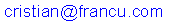# Mastermind

The game of mastermind is played as follows. One of the two players chooses a four digits number. All digits should be between 1 and 9, and no two digits should be the same, i.e. all four digits are distinct. The other player tries to guess the number. He proposes a number, and the first player answers with two numbers, the number of fixed digits, and the number of floppy digits. The number of fixed digits is the number of digits in the number that are correct and in place. The number of floppy digits is the number of digits that are correct, but not in place. For example, if the real number is 1493, and the guess is 4398, then there is one fixed digit, 9, and 2 floppy digits, 3 and 4.

Player two keeps proposing numbers until s/he guesses the number. the number of tries is counted, then players exchange roles. The winner is the player with less tries after several games.

The following program plays the role of the guesser. Just choose a number and press "Restart", and the computer will ask questions until it guesses the number you had in mind. If you can make it ask more than 6 questions, please let me know at. Enjoy! You can download the sources here.